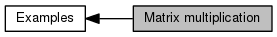Cyclops Tensor Framework parallel arithmetic on multidimensional arrays
Matrix multiplication

Multiplication of two matrices with user-defined attributes of symmetry and sparsity. More...

Collaboration diagram for Matrix multiplication:## Functions

int matmul (int m, int n, int k, World &dw, int sym_A=NS, int sym_B=NS, int sym_C=NS, double sp_A=1., double sp_B=1., double sp_C=1., bool test=true, bool bench=false, int niter=10)
(if test) tests and (if bench) benchmarks m*n*k matrix multiplication with matrices of specified symmetry and sparsity fraction More...

char * getCmdOption (char **begin, char **end, const std::string &option)

int main (int argc, char **argv)

## Detailed Description

Multiplication of two matrices with user-defined attributes of symmetry and sparsity.

## Function Documentation

 char* getCmdOption ( char ** begin, char ** end, const std::string & option )

Definition at line 162 of file matmul.cxx.

Referenced by main().

 int main ( int argc, char ** argv )

Definition at line 173 of file matmul.cxx.

References AS, getCmdOption(), matmul(), ctf.core::np(), NS, ctf.core::rank(), SH, and SY.

 int matmul ( int m, int n, int k, World & dw, int sym_A = `NS`, int sym_B = `NS`, int sym_C = `NS`, double sp_A = `1.`, double sp_B = `1.`, double sp_C = `1.`, bool test = `true`, bool bench = `false`, int niter = `10` )

(if test) tests and (if bench) benchmarks m*n*k matrix multiplication with matrices of specified symmetry and sparsity fraction

Parameters
 [in] m number of rows in C, A [in] n number of cols in C, B [in] k number of rows in A, cols in B [in] dw set of processors on which to execute matmul [in] sym_A in {NS, SY, AS, SH} symmetry attributes of A [in] sym_B in {NS, SY, AS, SH} symmetry attributes of B [in] sym_C in {NS, SY, AS, SH} symmetry attributes of C [in] sp_A fraction of nonzeros in A (if 1. A stored as dense) [in] sp_B fraction of nonzeros in B (if 1. B stored as dense) [in] sp_C fraction of nonzeros in C (if 1. C stored as dense) [in] test whether to test [in] bench whether to benchmark [in] niter how many iterations to compute

Definition at line 28 of file matmul.cxx.

Referenced by main().